Transfer learning is a powerful technique for training deep neural networks that allows one to take knowledge learned about one deep learning problem and apply it to a different, yet similar learning problem.

Using transfer learning can dramatically speed up the rate of deployment for an app you are designing, making both the training and implementation of your deep neural network simpler and easier.

In this article we'll go over the theory behind transfer learning and see how to carry out an example of transfer learning on Convolutional Neural Networks (CNNs) in PyTorch.

### What is PyTorch?

Pytorch is a library developed for Python, specializing in deep learning and natural language processing. PyTorch takes advantage of the power of Graphical Processing Units (GPUs) to make implementing a deep neural network faster than training a network on a CPU.

PyTorch has seen increasing popularity with deep learning researchers thanks to its speed and flexibility. PyTorch sells itself on three different features:

• A simple, easy-to-use interface
• Complete integration with the Python data science stack
• Flexible / dynamic computational graphs that can be changed during run time (which makes training a neural network significantly easier when you have no idea how much memory will be required for your problem).

PyTorch is compatible with NumPy and it allows NumPy arrays to be transformed into tensors and vice versa.

### Defining Necessary Terms

Before we go any further, let's take a moment to define some terms related to Transfer Learning. Getting clear on our definitions will make understanding of the theory behind transfer learning and implementing an instance of transfer learning easier to understand and replicate.

#### What is Deep Learning?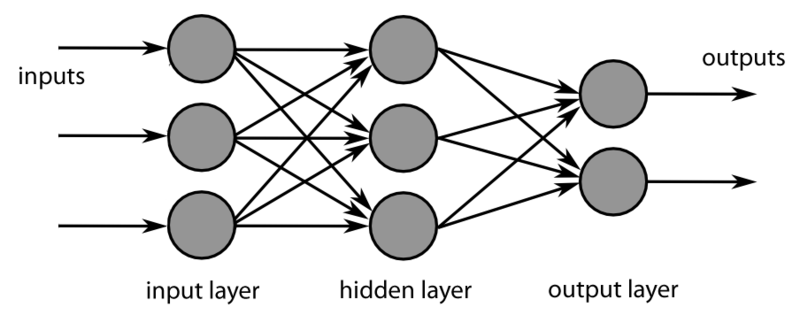Deep learning is a subsection of machine learning, and machine learning can be described as simply the act of enabling computers to carry out tasks without being explicitly programmed to do so.

Deep Learning systems utilize neural networks, which are computational frameworks modeled after the human brain.

Neural networks have three different components: An input layer, a hidden layer or middle layer, and an output layer.

The input layer is simply where the data that is being sent into the neural network is processed, while the middle layers/hidden layers are comprised of a structure referred to as a node or neuron.

These nodes are mathematical functions which alter the input information in some way and passes on the altered data to the final layer, or the output layer. Simple neural networks can distinguish simple patterns in the input data by adjusting the assumptions, or weights, about how the data points are related to one another.

A deep neural network gets its name from the fact that it is made out of many regular neural networks joined together. The more neural networks are linked together, the more complex patterns the deep neural network can distinguish and the more uses it has. There are different kinds of neural networks, which each type having its own specialty.

For example, Long Short Term Memory deep neural networks are networks that work very well when handling time sensitive tasks, where the chronological order of data is important, like text or speech data.

#### What is a Convolutional Neural Network?

This article will be concerned with Convolutional Neural Networks, a type of neural network that excels at manipulating image data.

Convolutional Neural Networks (CNNs) are special types of neural networks, adept at creating representations of visual data. The data in a CNN is represented as a grid which contains values that represent how bright, and what color, every pixel in the image is.

A CNN is broken down into three different components: the convolutional layers, the pooling layers, and the fully connected layers.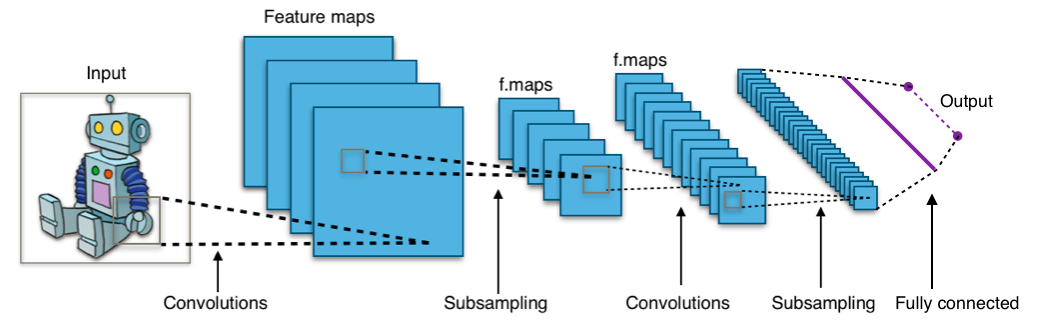The responsibility of the convolutional layer is to create a representation of the image by taking the dot product of two matrices.

The first matrix is a set of learnable parameters, referred to as a kernel. The other matrix is a portion of the image being analyzed, which will have a height, a width, and color channels. The convolutional layers are where the most computation happens in a CNN. The kernel is moved across the entire width and height of the image, eventually producing a representation of the entire image that is two-dimensional, a representation known as an activation map.

Due to the sheer amount of information contained in the CNN's convolutional layers, it can take an extremely long time to train the network. The function of the pooling layers is to reduce the amount of information contained in the CNNs convolutional layers, taking the output from one convolutional layer and scaling it down to make the representation simpler.

The pooling layer accomplishes this by looking at different spots in the network's outputs and "pooling" the nearby values, coming up with a single value that represents all the nearby values. In other words, it takes a summary statistic of the values in a chosen region.

Summarizing the values in a region means that the network can greatly reduce the size and complexity of its representation while still keeping the relevant information that will enable the network to recognize that information and draw meaningful patterns from the image.

There are various functions that can be used to summarize a region's values, such as taking the average of a neighborhood - or Average Pooling. A weighted average of the neighborhood can also be taken, as can the L2 norm of the region. The most common pooling technique is Max Pooling, where the maximum value of the region is taken and used to represent the neighborhood.

The fully connected layer is where all the neurons are linked together, with connections between every preceding and succeeding layer in the network. This is where the information that has been extracted by the convolutional layers and pooled by the pooling layers is analyzed, and where patterns in the data are learned. The computations here are carried out through matrix multiplication combined with a bias effect.

There are also several nonlinearities present in the CNN. When considering that images themselves are non-linear things, the network has to have nonlinear components to be able to interpret the image data. The nonlinear layers are usually inserted into the network directly after the convolutional layers, as this gives the activation map non-linearity.

There are a variety of different nonlinear activation functions that can be used for the purpose of enabling the network to properly interpret the image data. The most popular nonlinear activation function is ReLu, or the Rectified Linear Unit. The ReLu function turns nonlinear inputs into a linear representation by compressing real values to only positive values above 0. To put that another way, the ReLu function takes any value above zero and returns it as is, while if the value is below zero it is returned as zero.

The ReLu function is popular because of its reliability and speed, performing around six times faster than other activation functions. The downside to ReLu is that it can easily get stuck when handling large gradients, never updating the neurons. This problem can be tackled by setting a learning rate for the function.

Two other popular nonlinear functions are the sigmoid function and the Tanh function.

The sigmoid function works by taking real values and squishing them to a range between 0 and 1, although it has problems handling activations that are near the extremes of the gradient, as the values become almost zero.

Meanwhile, the Tanh function operates similarly to the Sigmoid, except that its output is centered near zero and it squishes the values to between -1 and 1.

#### Training and Testing

There are two different phases to creating and implementing a deep neural network: training and testing.

The training phase is where the network is fed the data and it begins to learn the patterns that the data contains, adjusting the weights of the network, which are assumptions about how the data points are related to each other. To put that another way, the training phase is where the network "learns" about the data is has been fed.

The testing phase is where what the network has learned is evaluated. The network is given a new set of data, one it hasn't seen before, and then the network is asked to apply its guesses about the patterns it has learned to the new data. The accuracy of the model is evaluated and typically the model is tweaked and retrained, then retested, until the architect is satisfied with the model's performance.

In the case of transfer learning, the network that is used has been pretrained. The network's weights have already been adjusted and saved, so there's no reason to train the entire network again from scratch. This means that the network can immediately be used for testing, or just certain layers of the network can be tweaked and then retrained. This greatly speeds up the deployment of the deep neural network.

#### What is Transfer Learning?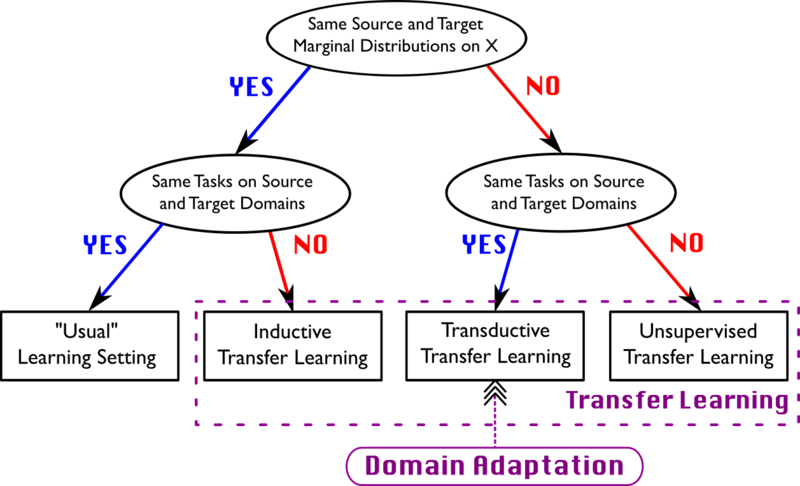The idea behind transfer learning is taking a model trained on one task and applying to a second, similar task. The fact that a model has already had some or all of the weights for the second task trained means that the model can be implemented much quicker. This allows rapid performance assessment and model tuning, enabling quicker deployment overall. Transfer learning is becoming increasingly popular in the field of deep learning, thanks to the vast amount of computational resources and time needed to train deep learning models, in addition to large, complex datasets.

The primary constraint of transfer learning is that the model features learned during the first task are general, and not specific to the first task. In practice, this means that models trained to recognize certain types of images can be reused to recognize other images, as long as the general features of the images are similar.

### Transfer Learning Theory

The utilization of transfer learning has several important concepts. In order to understand the implementation of transfer learning, we need go over what a pre-trained model looks like, and how that model can be fine-tuned for your needs.

There are two ways to choose a model for transfer learning. It is possible to create a model from scratch for your own needs, save the model's parameters and structure, and then reuse the model later.

The second way to implement transfer learning is to simply take an already existing model and reuse it, tuning its parameters and hyperparameters as you do so. In this instance, we will be using a pretrained model and modifying it. After you've decided what approach you want to use, choose a model (if you are using a pretrained model).

There is a large variety of pretrained models that can be used in PyTorch. Some of the pretrained CNNs include:

• AlexNet
• CaffeResNet
• Inception
• The ResNet series
• The VGG series

These pretrained models are accessible through PyTorch's API and when instructed, PyTorch will download their specifications to your machine. The specific model we are going to be using is ResNet34, part of the Resnet series.

The Resnet model was developed and trained on an ImageNet dataset as well as the CIFAR-10 dataset. As such it is optimized for visual recognition tasks, and showed a marked improvement over the VGG series, which is why we will be using it.

However, other pretrained models exist, and you may want to experiment with them to see how they compare.

As PyTorch's documentation on transfer learning explains, there are two major ways that transfer learning is used: fine-tuning a CNN or by using the CNN as a fixed feature extractor.

When fine-tuning a CNN, you use the weights the pretrained network has instead of randomly initializing them, and then you train like normal. In contrast, a feature extractor approach means that you'll maintain all the weights of the CNN except for those in the final few layers, which will be initialized randomly and trained as normal.

Fine-tuning a model is important because although the model has been pretrained, it has been trained on a different (though hopefully similar) task. The densely connected weights that the pretrained model comes with will probably be somewhat insufficient for your needs, so you will likely want to retrain the final few layers of the network.

In contrast, because the first few layers of the network are just feature extraction layers, and they will perform similarly on similar images, they can be left as they are. Therefore, if the dataset is small and similar, the only training that needs to be done is the training of the final few layers. The larger and more complex the dataset gets, the more the model will need to be retrained. Remember that transfer learning works best when the dataset you are using is smaller than the original pre-trained model, and similar to the images fed to the pretrained model.

Working with transfer learning models in Pytorch means choosing which layers to freeze and which to unfreeze. Freezing a model means telling PyTorch to preserve the parameters (weights) in the layers you've specified. Unfreezing a model means telling PyTorch you want the layers you've specified to be available for training, to have their weights trainable.

After you've concluded training your chosen layers of the pretrained model, you'll probably want to save the newly trained weights for future use. Even though using a pre-trained models is faster than and training a model from scratch, it still takes time to train, so you'll want to copy the best model weights.

### Image Classification with Transfer Learning in PyTorch

We're ready to start implementing transfer learning on a dataset. We'll cover both finetuning the ConvNet and using the net as a fixed feature extractor.

#### Data Preprocessing

First off, we'll need to decide on a dataset to use. Let's choose something that has a lot of really clear images to train on. The Stanford Cats and Dogs dataset is a very commonly used dataset, chosen for how simple yet illustrative the set is. You can download this right here.

Be sure to divide the dataset into two equally sized sets: "train" and "val".

You can do this anyway that you would like, by manually moving the files or by writing a function to handle it. You may also want to limit the dataset to a smaller size, as it comes with almost 12,000 images in each category, and this will take a long time to train. You may want to cut that number down to around 5000 in each category, with 1000 set aside for validation. However, the number of images you want to use for training is up to you.

Here's one way to prepare the data for use:

``````import os
import shutil
import re base_dir = "PetImages/" # Create training folder
files = os.listdir(base_dir) # Moves all training cat images to cats folder, training dog images to dogs folder
def train_maker(name): train_dir = f"{base_dir}/train/{name}" for f in files: search_object = re.search(name, f) if search_object: shutil.move(f'{base_dir}/{name}', train_dir) train_maker("Cat")
train_maker("Dog") # Make the validation directories
try: os.makedirs("val/Cat") os.makedirs("val/Dog")
except OSError: print ("Creation of the directory %s failed")
else: print ("Successfully created the directory %s ") # Create validation folder cat_train = base_dir + "train/Cat/"
cat_val = base_dir + "val/Cat/"
dog_train = base_dir + "train/Dog/"
dog_val = base_dir + "val/Dog/" cat_files = os.listdir(cat_train)
dog_files = os.listdir(dog_train) # This will put 1000 images from the two training folders
# into their respective validation folders for f in cat_files: validationCatsSearchObj = re.search("5\d\d\d", f) if validationCatsSearchObj: shutil.move(f'{cat_train}/{f}', cat_val) for f in dog_files: validationCatsSearchObj = re.search("5\d\d\d", f) if validationCatsSearchObj: shutil.move(f'{dog_train}/{f}', dog_val)
``````

After we have selected and prepared the data, we can start off by importing all the necessary libraries. We'll need many of the Torch packages like `nn` neural network, the optimizers and the `DataLoaders`. We'll also want `matplotlib` to visualize some of our training examples.

We need `numpy` to handle the creation of data arrays, as well as a few other miscellaneous modules:

``````from __future__ import print_function, division import torch
import torch.nn as nn
import torch.optim as optim
from torch.optim import lr_scheduler
import torchvision
from torchvision import datasets, models, transforms
import matplotlib.pyplot as plt
import numpy as np
import time
import os
import copy
``````

To start off with, we need to load in our training data and prepare it for use by our neural network. We're going to be making use of Pytorch's `transforms` for that purpose. We'll need to make sure the images in the training set and validation set are the same size, so we'll be using `transforms.Resize`.

We'll also be doing a little data augmentation, trying to improve the performance of our model by forcing it to learn about images at different angles and crops, so we'll randomly crop and rotate the images.

Next, we'll make tensors out of the images, as PyTorch works with tensors. Finally, we'll normalize the images, which helps the network work with values that may be have a wide range of different values.

We then `compose` all our chosen transforms. Note that the validation transforms don't have any of the flipping or rotating, as they aren't part of our training set, so the network isn't learning about them:

``````# Make transforms and use data loaders # We'll use these a lot, so make them variables
mean_nums = [0.485, 0.456, 0.406]
std_nums = [0.229, 0.224, 0.225] chosen_transforms = {'train': transforms.Compose([ transforms.RandomResizedCrop(size=256), transforms.RandomRotation(degrees=15), transforms.RandomHorizontalFlip(), transforms.ToTensor(), transforms.Normalize(mean_nums, std_nums)
]), 'val': transforms.Compose([ transforms.Resize(256), transforms.CenterCrop(224), transforms.ToTensor(), transforms.Normalize(mean_nums, std_nums)
]),
}
``````

Now we will set the directory for our data and use PyTorch's `ImageFolder` function to create datasets:

``````# Set the directory for the data
data_dir = '/data/' # Use the image folder function to create datasets
chosen_datasets = {x: datasets.ImageFolder(os.path.join(data_dir, x), chosen_transforms[x]) for x in ['train', 'val']}
``````

Now that we have chosen the image folders we want, we need to use the DataLoaders to create iterable objects for us to work with. We tell it which datasets we want to use, give it a batch size, and shuffle the data.

``````# Make iterables with the dataloaders
dataloaders = {x: torch.utils.data.DataLoader(chosen_datasets[x], batch_size=4, shuffle=True, num_workers=4) for x in ['train', 'val']}
``````

We're going to need to preserve some information about our dataset, specifically the size of the dataset and the names of the classes in our dataset. We also need to specify what kind of device we are working with, a CPU or GPU. The following setup will use GPU if available, otherwise CPU will be used:

``````dataset_sizes = {x: len(chosen_datasets[x]) for x in ['train', 'val']}
class_names = chosen_datasets['train'].classes device = torch.device("cuda" if torch.cuda.is_available() else "cpu")
``````

Now let's try visualizing some of our images with a function. We'll take an input, create a Numpy array from it, and transpose it. Then we'll normalize the input using mean and standard deviation. Finally, we'll clip values to between 0 and 1 so there isn't a massive range in the possible values of the array, and then show the image: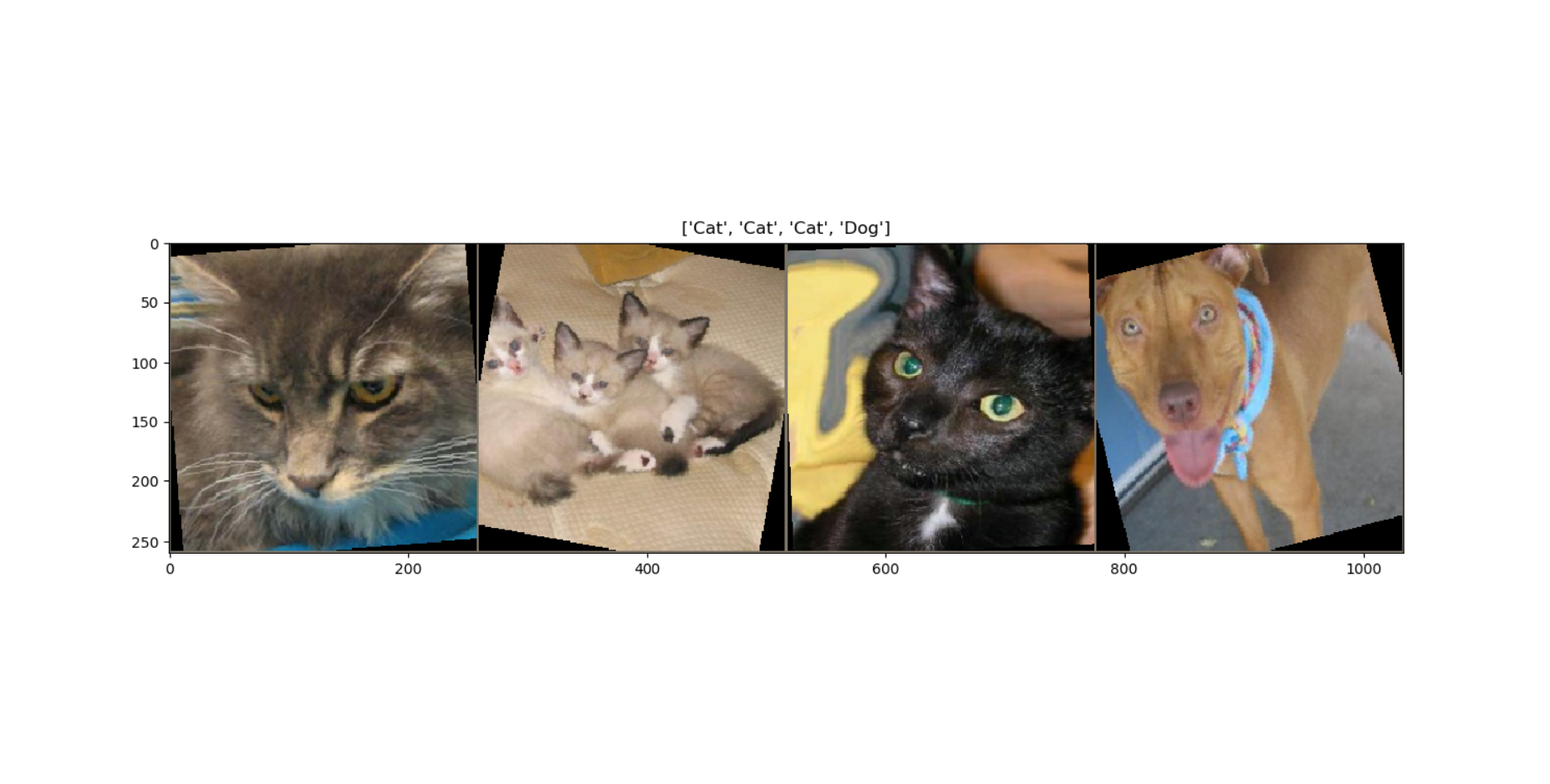``````# Visualize some images
def imshow(inp, title=None): inp = inp.numpy().transpose((1, 2, 0)) mean = np.array([mean_nums]) std = np.array([std_nums]) inp = std * inp + mean inp = np.clip(inp, 0, 1) plt.imshow(inp) if title is not None: plt.title(title) plt.pause(0.001) # Pause a bit so that plots are updated
``````

Now let's use that function and actually visualize some of the data. We're going to get the inputs and the name of the classes from the `DataLoader` and store them for later use. Then we'll make a grid to display the inputs on and display them:

``````# Grab some of the training data to visualize
inputs, classes = next(iter(dataloaders['train'])) # Now we construct a grid from batch
out = torchvision.utils.make_grid(inputs) imshow(out, title=[class_names[x] for x in classes])
``````

#### Setting up a Pretrained Model

Now we have to set up the pretrained model we want to use for transfer learning. In this case, we're going to use the model as is and just reset the final fully connected layer, providing it with our number of features and classes.

When using pretrained models, PyTorch sets the model to be unfrozen (will have its weights adjusted) by default. So we'll be training the whole model:

``````# Setting up the model
# load in pretrained and reset final fully connected res_mod = models.resnet34(pretrained=True) num_ftrs = res_mod.fc.in_features
res_mod.fc = nn.Linear(num_ftrs, 2)
``````

If this still seems somewhat unclear, visualizing the composition of the model may help.

``````for name, child in res_mod.named_children(): print(name)
``````

Here's what that returns:

``````conv1
bn1
relu
maxpool
layer1
layer2
layer3
layer4
avgpool
fc
``````

Notice the final portion is `fc`, or "Fully-Connected". This is the only layer we are modifying the shape of, giving it our two classes to output.

Essentially, we're going to be changing the outputs of the final fully connected portion to just two classes, and adjusting the weights for all the other layers.

Now we need to send our model to our training device. We also need to choose the loss criterion and optimizer we want to use with the model. `CrossEntropyLoss` and the `SGD` optimizer are good choices, though there are many others.

We'll also be choosing a learning rate scheduler, which decreases the learning rate of the optimizer overtime and helps prevent nonconvergence due to large learning rates. You can learn more about learning rate schedulers here if you are curious:

``````res_mod = res_mod.to(device)
criterion = nn.CrossEntropyLoss() # Observe that all parameters are being optimized
optimizer_ft = optim.SGD(res_mod.parameters(), lr=0.001, momentum=0.9) # Decay LR by a factor of 0.1 every 7 epochs
exp_lr_scheduler = lr_scheduler.StepLR(optimizer_ft, step_size=7, gamma=0.1)
``````

Now we just need to define the functions that will train the model and visualize the predictions.

Let's start off with the training function. It will take in our chosen model as well as the optimizer, criterion, and scheduler we chose. We'll also specify a default number of training epochs.

Every epoch will have a training and validation phase. To begin with, we set the model's initial best weights to those of the pretrained mode, by using `state_dict`.

Now, for every epoch in the chosen number of epochs, if we are in the training phase, we will:

1. Decrement the learning rate
3. Carry out the forward training pass
4. Calculate the loss
5. Do backward propagation and update the weights with the optimizer

We'll also be keeping track of the model's accuracy during the training phase, and if we move to the validation phase and the accuracy has improved, we'll save the current weights as the best model weights:

``````def train_model(model, criterion, optimizer, scheduler, num_epochs=10): since = time.time() best_model_wts = copy.deepcopy(model.state_dict()) best_acc = 0.0 for epoch in range(num_epochs): print('Epoch {}/{}'.format(epoch, num_epochs - 1)) print('-' * 10) # Each epoch has a training and validation phase for phase in ['train', 'val']: if phase == 'train': scheduler.step() model.train() # Set model to training mode else: model.eval() # Set model to evaluate mode current_loss = 0.0 current_corrects = 0 # Here's where the training happens print('Iterating through data...') for inputs, labels in dataloaders[phase]: inputs = inputs.to(device) labels = labels.to(device) # We need to zero the gradients, don't forget it optimizer.zero_grad() # Time to carry out the forward training poss # We only need to log the loss stats if we are in training phase with torch.set_grad_enabled(phase == 'train'): outputs = model(inputs) _, preds = torch.max(outputs, 1) loss = criterion(outputs, labels) # backward + optimize only if in training phase if phase == 'train': loss.backward() optimizer.step() # We want variables to hold the loss statistics current_loss += loss.item() * inputs.size(0) current_corrects += torch.sum(preds == labels.data) epoch_loss = current_loss / dataset_sizes[phase] epoch_acc = current_corrects.double() / dataset_sizes[phase] print('{} Loss: {:.4f} Acc: {:.4f}'.format( phase, epoch_loss, epoch_acc)) # Make a copy of the model if the accuracy on the validation set has improved if phase == 'val' and epoch_acc > best_acc: best_acc = epoch_acc best_model_wts = copy.deepcopy(model.state_dict()) print() time_since = time.time() - since print('Training complete in {:.0f}m {:.0f}s'.format( time_since // 60, time_since % 60)) print('Best val Acc: {:4f}'.format(best_acc)) # Now we'll load in the best model weights and return it model.load_state_dict(best_model_wts) return model
``````

Our training printouts should look something like this:

``````Epoch 0/25
----------
Iterating through data...
train Loss: 0.5654 Acc: 0.7090
Iterating through data...
val Loss: 0.2726 Acc: 0.8889 Epoch 1/25
----------
Iterating through data...
train Loss: 0.5975 Acc: 0.7090
Iterating through data...
val Loss: 0.2793 Acc: 0.8889 Epoch 2/25
----------
Iterating through data...
train Loss: 0.5919 Acc: 0.7664
Iterating through data...
val Loss: 0.3992 Acc: 0.8627
``````

#### Visualization

Now we'll create a function that will let us see the predictions our model has made.

``````def visualize_model(model, num_images=6): was_training = model.training model.eval() images_handeled = 0 fig = plt.figure() with torch.no_grad(): for i, (inputs, labels) in enumerate(dataloaders['val']): inputs = inputs.to(device) labels = labels.to(device) outputs = model(inputs) _, preds = torch.max(outputs, 1) for j in range(inputs.size()): images_handeled += 1 ax = plt.subplot(num_images//2, 2, images_handeled) ax.axis('off') ax.set_title('predicted: {}'.format(class_names[preds[j]])) imshow(inputs.cpu().data[j]) if images_handeled == num_images: model.train(mode=was_training) return model.train(mode=was_training)
``````

Now we can tie everything together. We'll train the model on our images and show the predictions: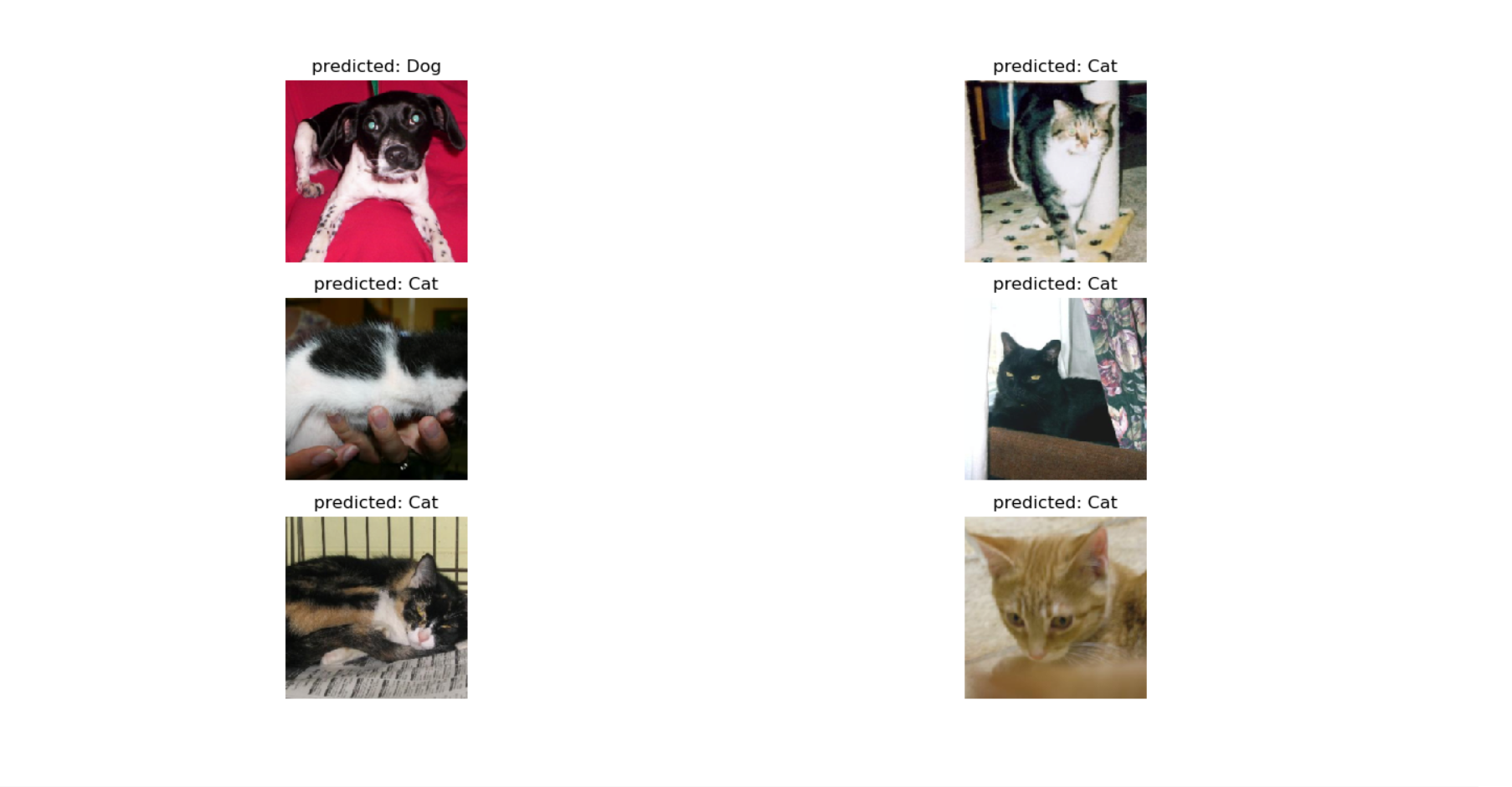``````base_model = train_model(res_mod, criterion, optimizer_ft, exp_lr_scheduler, num_epochs=3)
visualize_model(base_model)
plt.show()
``````

That training will probably take you a long while if you are using a CPU and not a GPU. It will still take some time even if using a GPU.

It is due to the long training time that many people choose to simply use the pretrained model as a fixed feature extractor, and only train the last layer or so. This significantly speeds up training time. In order to do that, you'll need to replace the model we've built. There will be a link to a GitHub repo for both versions of the ResNet implementation.

Replace the section where the pretrained model is defined with a version that freezes the weights and doesn't carry our gradient calculations or backprop.

It looks quite similar to before, except that we specify that the gradients don't need computation:

``````# Setting up the model
# Note that the parameters of imported models are set to requires_grad=True by default res_mod = models.resnet34(pretrained=True)
for param in res_mod.parameters(): param.requires_grad = False num_ftrs = res_mod.fc.in_features
res_mod.fc = nn.Linear(num_ftrs, 2) res_mod = res_mod.to(device)
criterion = nn.CrossEntropyLoss() # Here's another change: instead of all paramters being optimized
# only the params of the final layers are being optmized optimizer_ft = optim.SGD(res_mod.fc.parameters(), lr=0.001, momentum=0.9) exp_lr_scheduler = lr_scheduler.StepLR(optimizer_ft, step_size=7, gamma=0.1)
``````

What if we wanted to selectively unfreeze layers and have the gradients computed for just a few chosen layers. Is that possible? Yes, it is.

Let's print out the children of the model again to remember what layers/components it has:

``````for name, child in res_mod.named_children(): print(name)
``````

Here's the layers:

``````conv1
bn1
relu
maxpool
layer1
layer2
layer3
layer4
avgpool
fc
``````

Now that we know what the layers are, we can unfreeze ones we want, like just layers 3 and 4:

``````for name, child in res_mod.named_children(): if name in ['layer3', 'layer4']: print(name + 'has been unfrozen.') for param in child.parameters(): param.requires_grad = True else: for param in child.parameters(): param.requires_grad = False
``````

Of course, we'll also need to update the optimizer to reflect the fact that we only want to optimize certain layers.

``````optimizer_conv = torch.optim.SGD(filter(lambda x: x.requires_grad, res_mod.parameters()), lr=0.001, momentum=0.9)
``````

So now you know that you can tune the entire network, just the last layer, or something in between.

### Conclusion

Congratulations, you've now implemented transfer learning in PyTorch. It would be a good idea to compare the implementation of a tuned network with the use of a fixed feature extractor to see how the performance differs. Experimenting with freezing and unfreezing certain layers is also encouraged, as it lets you get a better sense of how you can customize the model to fit your needs.

Here's some other things you can try:

• Using different pretrained models to see which ones perform better under different circumstances
• Changing some of the arguments of the model, like adjusting learning rate and momentum
• Try classification on a dataset with more than two classes

If you're curious to learn more about different transfer learning applications and the theory behind it, there's an excellent breakdown of some of the math behind it as well as use cases
here.

The code for this article can be found in this GitHub repo.